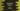# 4 ways in TypeScript to reverse a string## How to reverse a string in TypeScript:

In this post, we will learn how to reverse a string in TypeScript. It is a common interview question and also a common problem in software development. There are different ways available to reverse a string in TypeScript and JavaScript. In this post, I will show you how these methods work with examples.

### Method 1: Reverse a string by using a for loop:

This is the naive approach to reverse a string. We will use one for loop that will iterate over the characters of the string one by one in reverse order and build the reversed string.

It will use the following algorithm:

• Take the string as input from the user.
• Initialize one variable as an empty string to hold the result.
• Iterate over the characters of the string from end to start.
• Add each character to the result string.
• At the end of the program, print the result string.

Below is the complete program:

``````function reverseString(str: string) {
let result = "";
for (let i = str.length - 1; i >= 0; i--) {
result += str[i];
}
return result;
}

let given_str = "hello world";
let reverse_str = reverseString(given_str);

console.log("Given string: " + given_str);
console.log("Reversed string: " + reverse_str);``````

In this program, the reverseString method is used to reverse the string. This method takes one string as the parameter and returns the reversed string.

• The variable result is initialized as an empty string to hold the final reverse string.
• It uses a for loop to iterate over the characters from end to start of the string. The loop runs with variable i from the index of the last character to the index of the first character.
• On each iteration, it appends the current iterating character to the end of result.
• Once the loop completes, the result variable holds the reverse string. It returns this value to the caller.

If you run this program, it will print the below output:

``````Given string: hello world
Reversed string: dlrow olleh``````

### Method 2: Reverse a string by using a while loop:

Another way to reverse a string in Typescript is by using a while loop. It is similar to the above program. The only difference will be the loop.

``````function reverseString(str: string) {
let result = "";
let i = str.length - 1;

while (i >= 0) {
result += str[i];
i--;
}
return result;
}

let given_str = "hello world";
let reverse_str = reverseString(given_str);

console.log("Given string: " + given_str);
console.log("Reversed string: " + reverse_str);``````

The output of the program will be similar to the first example.

### Method 3: By splitting the string:

We don’t have any reverse method for TypeScript/JavaScript strings. But, we have one method to get an array of all the characters of the string and we can reverse the array content by using the reverse() method and join these characters to create a string.

Following are the methods we will use:

1. split() to split the string into sub-strings. This method takes one parameter and breaks the string at the passed parameter. If we pass an empty string as the parameter, it will return one array holding its characters.
2. The reverse() method of array reverses the content of an array in place.
3. The join() method of array joins the array elements and creates one string. We have to pass an empty string as its parameter, otherwise, it will use commas to join the sub-strings.

So, we have to split, reverse and join a string to reverse it.

Let me show you the program:

``````function reverseString(str: string) {
let strArr = str.split("");
let reverseArr = strArr.reverse();
let reverseStr = reverseArr.join('');
return reverseStr;
}

let given_str = "hello world";
let reverse_str = reverseString(given_str);

console.log("Given string: " + given_str);
console.log("Reversed string: " + reverse_str);``````

Here, strArr is the array of characters, reverseArr is the reverse array and reverseStr is the final reverse string.

We can also write this in one line.

``````function reverseString(str: string) {
return str.split("").reverse().join("");
}

let given_str = "hello world";
let reverse_str = reverseString(given_str);

console.log("Given string: " + given_str);
console.log("Reversed string: " + reverse_str);``````

It will print the same output.

### Method 4: Recursive method:

Another way to solve this is by using a recursive method. Below program finds the reverse string recursively:

``````function reverseString(s: string) {
if (s === "") return "";

return reverseString(s.substring(1)) + s;
}

let given_str = "hello world";
let reverse_str = reverseString(given_str);

console.log("Given string: " + given_str);
console.log("Reversed string: " + reverse_str);``````

The if block checks if the string is an empty string. This is to stop the recursion. It picks the first character of the string and appends it to the end. Also, it calls the reverseString method recursively by taking the substring from the first character to the end.

It will give a similar result.